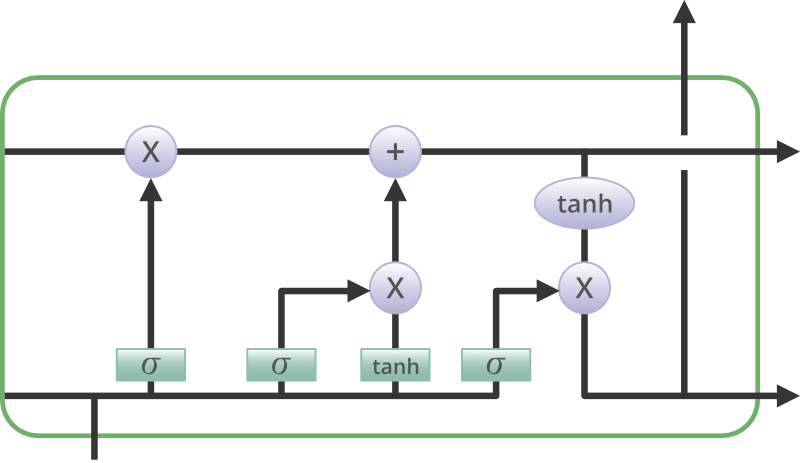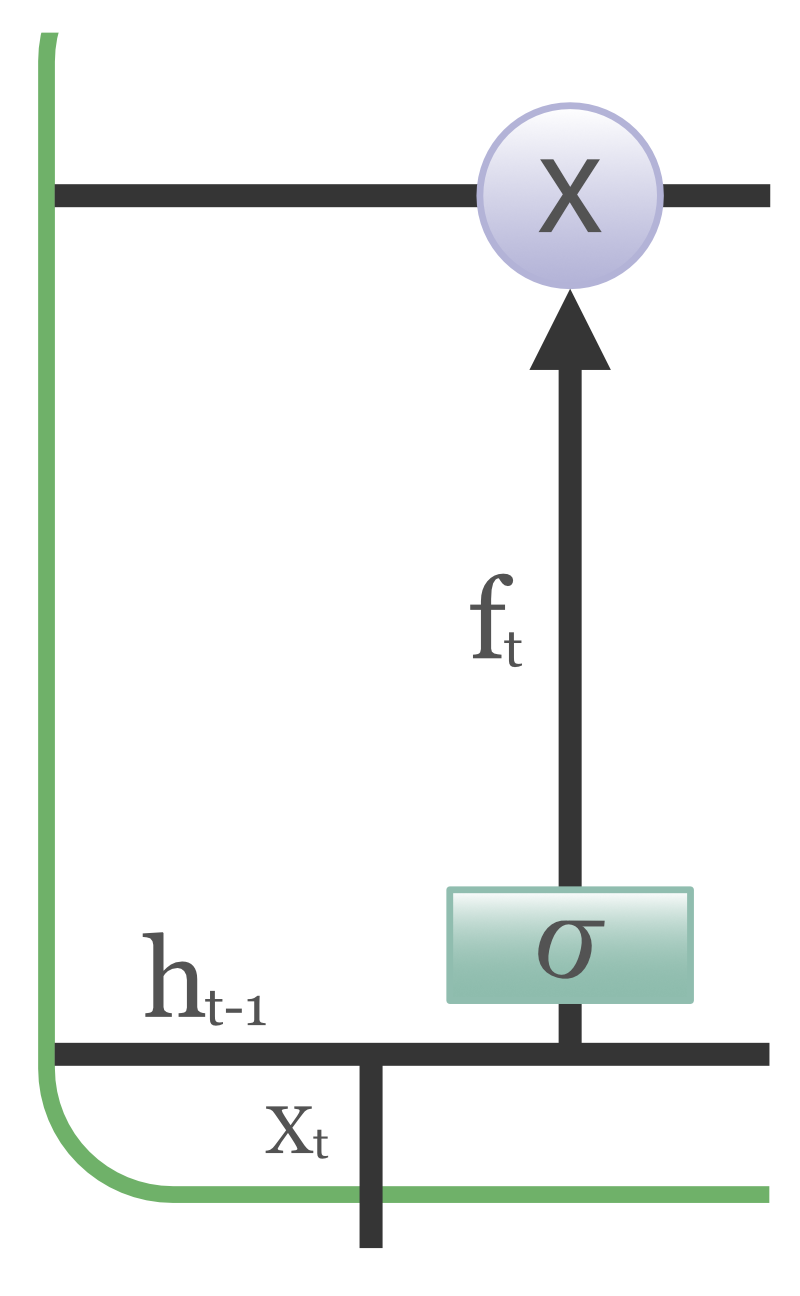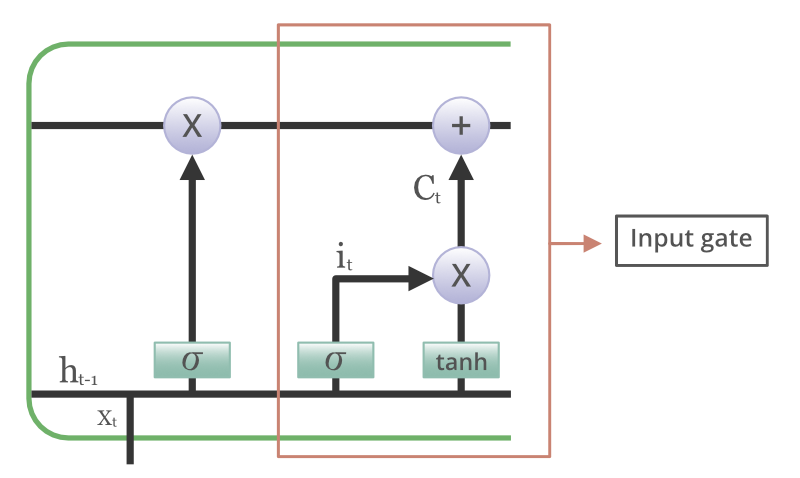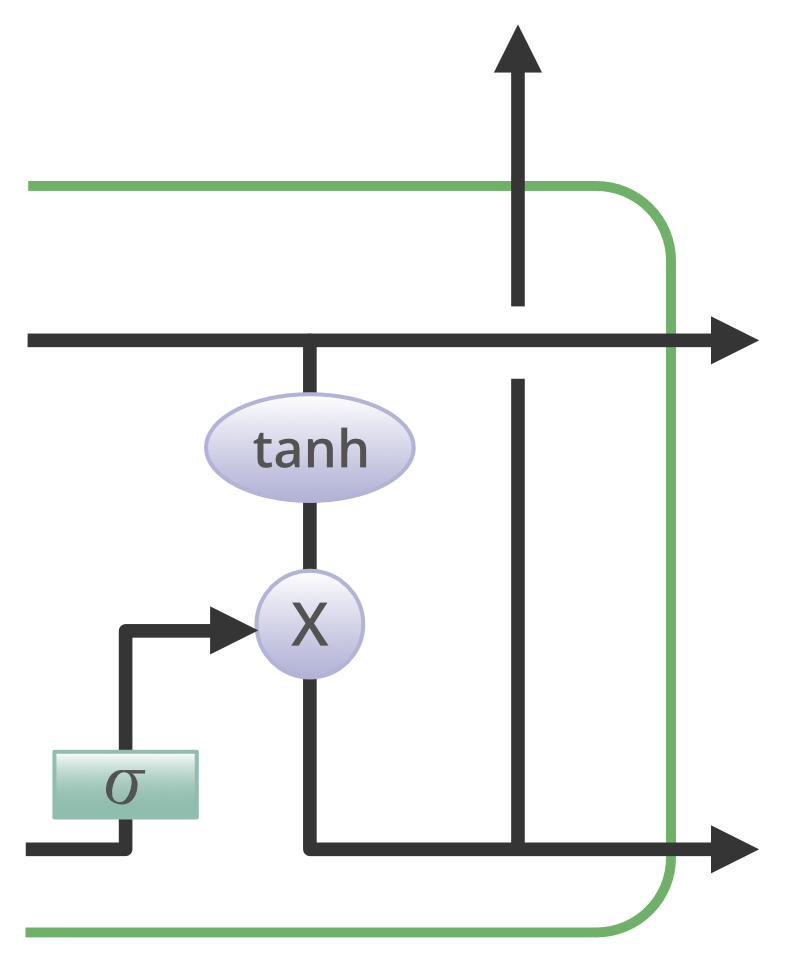# Deep Learning | Introduction to Long Short Term Memory

• Difficulty Level : Easy
• Last Updated : 29 Sep, 2021

Long Short Term Memory is a kind of recurrent neural network. In RNN output from the last step is fed as input in the current step. LSTM was designed by Hochreiter & Schmidhuber. It tackled the problem of long-term dependencies of RNN in which the RNN cannot predict the word stored in the long-term memory but can give more accurate predictions from the recent information. As the gap length increases RNN does not give an efficient performance. LSTM can by default retain the information for a long period of time. It is used for processing, predicting, and classifying on the basis of time-series data.

### Structure Of LSTM:

LSTM has a chain structure that contains four neural networks and different memory blocks called cells

Attention reader! Don’t stop learning now. Get hold of all the important Machine Learning Concepts with the Machine Learning Foundation Course at a student-friendly price and become industry ready.Information is retained by the cells and the memory manipulations are done by the gates. There are three gates –

1. Forget Gate: The information that is no longer useful in the cell state is removed with the forget gate. Two inputs x_t (input at the particular time) and h_t-1 (previous cell output) are fed to the gate and multiplied with weight matrices followed by the addition of bias. The resultant is passed through an activation function which gives a binary output. If for a particular cell state the output is 0, the piece of information is forgotten and for output 1, the information is retained for future use.2. Input gate: The addition of useful information to the cell state is done by the input gate. First, the information is regulated using the sigmoid function and filter the values to be remembered similar to the forget gate using inputs h_t-1 and x_t. Then, a vector is created using tanh function that gives an output from -1 to +1, which contains all the possible values from h_t-1 and x_t. At last, the values of the vector and the regulated values are multiplied to obtain the useful information3. Output gate: The task of extracting useful information from the current cell state to be presented as output is done by the output gate. First, a vector is generated by applying tanh function on the cell. Then, the information is regulated using the sigmoid function and filter by the values to be remembered using inputs h_t-1 and x_t. At last, the values of the vector and the regulated values are multiplied to be sent as an output and input to the next cell.Some of the famous applications of LSTM includes:

1. Language Modelling
2. Machine Translation
3. Image Captioning
4. Handwriting generation